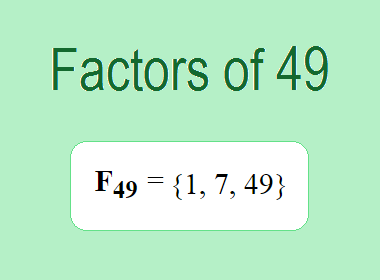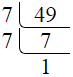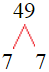# Factors of 49The factors of 49 are 1, 7, and 49 i.e. F49 = {1, 7, 49}. The factors of 49 are all the numbers that can divide 49 without leaving a remainder.

We can check if these numbers are factors of 49 by dividing 49 by each of them. If the result is a whole number, then the number is a factor of 49. Let's do this for each of the numbers listed above:

·        1 is a factor of 49 because 49 divided by 1 is 49.

·        7 is a factor of 49 because 49 divided by 7 is 7.

·        49 is a factor of 49 because 49 divided by 49 is 1.

## How to Find Factors of 49?

1 and the number itself are the factors of every number. So, 1 and 49 are two factors of 49. To find the other factors of 49, we can start by dividing 49 by the numbers between 1 and 49. If we divide 49 by 2, we get a remainder of 1. Therefore, 2 is not a factor of 49. If we divide 49 by 3, we get a remainder of 1. Therefore, 3 is not a factor of 49.

Next, we can check if 4 is a factor of 49. If we divide 49 by 4, we get a remainder of 1. Therefore, 4 is not a factor of 49. Similarly, If we divide 49 by 7, we get a remainder of 0. Therefore, 7 is a factor of 49. We can continue this process for all the possible factors of 49.

Through this process, we can find that the factors of 49 are 1, 7, and 49. These are the only numbers that can divide 49 without leaving a remainder.

********************

********************

********************

## Properties of the Factors of 49

The factors of 49 have some interesting properties. One of the properties is that the sum of the factors of 49 is equal to 57. We can see this by adding all the factors of 49 together:

1 + 7 + 49 = 57

Another property of the factors of 49 is that they are all odd numbers. This is because 49 is an odd number, and any even number cannot divide an odd number without leaving a remainder.

Another property of the factors of 49 is that the only prime factor of 49 is 7.

## Applications of the Factors of 49

The factors of 49 have several applications in mathematics. One of the applications is in finding the highest common factor (HCF) of two or more numbers. The HCF is the largest factor that two or more numbers have in common. For example, to find the HCF of 49 and 21, we need to find the factors of both numbers and identify the largest factor they have in common. The factors of 49 are 1, 7, and 49. The factors of 21 are 1, 3, 7, and 21. The largest factor that they have in common is 7. Therefore, the HCF of 49 and 21 is 7.

Another application of the factors of 49 is in prime factorization. Prime factorization is the process of expressing a number as the product of its prime factors. The only prime factor of 49 is 7. We can express 49 as:

49 = 7 × 7

We can do prime factorization by division and factor tree method also. Here is the prime factorization of 49 by division method,49 = 7 × 7

Here is the prime factorization of 49 by the factor tree method,49 = 7 × 7

## Conclusion

The factors of 49 are the numbers that can divide 49 without leaving a remainder. The factors of 49 are 1, 7, and 49. The factors of 49 have some interesting properties, such as being odd numbers and having a sum of 57. The factors of 49 have several applications in mathematics, such as finding the highest common factor and prime factorization.